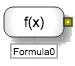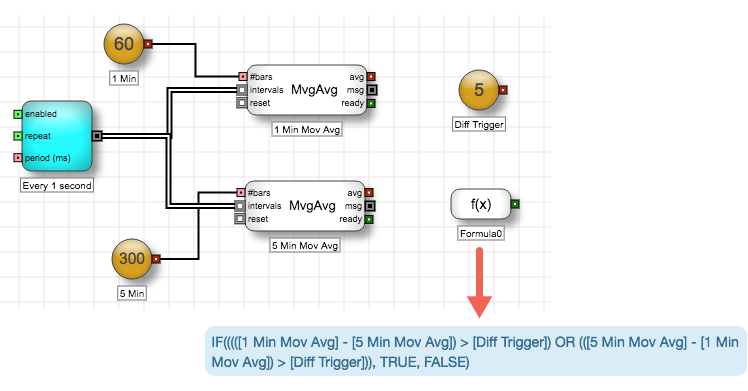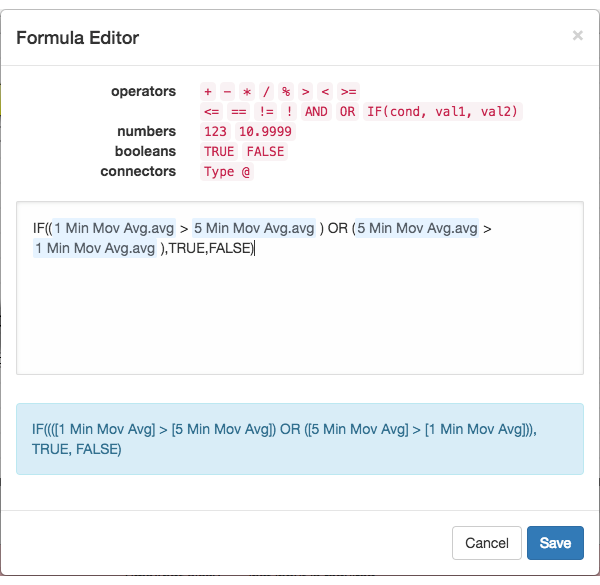# Arithmetic blocks

Arithmetic blocks

# Formula blockThe Formula block evaluates a user-defined equation and outputs the result of the evaluation as a True/False, numeric, or an instrument type output. The block recalculates and outputs its value whenever any of the connectors referenced in its internal formula changes. Any connector used in the formula establishes an implicit connection to the Formula block

Example The Formula block uses the avg outputs of the two Moving Average blocks to output TRUE when the difference between the 1-min and 5-min moving average exceeds the value of the Diff Trigger Number block. The Formula block outputs FALSE, otherwise.The formula for the block is specified as follows in the Formula Editor.In this example, the implicit connections from the two Moving Average block avg output ports cause the Formula block to recalculate when either of the avg output port values change.

## Block properties

To customize the block, update the Block Properties.

Property Description
Name Name to display beneath the block on the ADL canvas
Formula

Equation used to calculate the value of the block

If not yet defined, the field displays a Click to edit formula link that opens the Formula Builder.